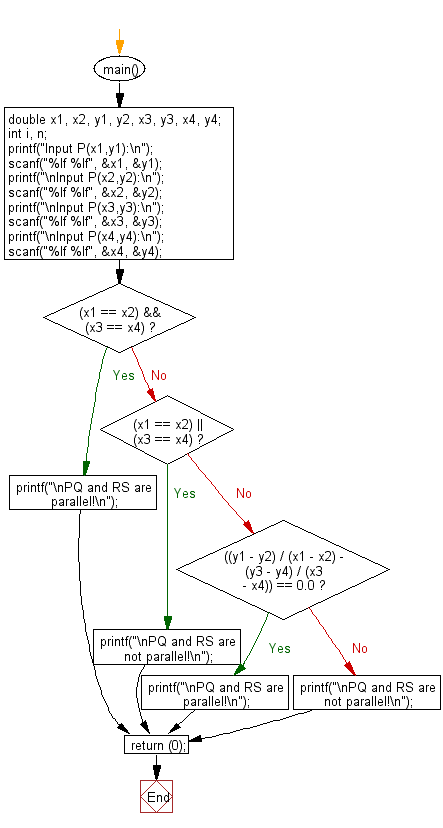﻿ C Program: Check two lines are parallel or not - w3resource

# C Exercises: Check two lines are parallel or not

## C Basic Declarations and Expressions: Exercise-138 with Solution

Write a C program to test whether two lines are parallel or not. The four points are P(x1, y1), Q(x2, y2), R(x3, y3) and S(x4, y4), check PQ and RS are parallel are not.

Input:
−100 <= x1, y1, x2, y2, x3, y3, x4, y4 <= 100
Each value is a real number with at most 5 digits after the decimal point.

Sample Solution:

C Code:

``````#include <stdio.h>

main() {
// Variable declarations
double x1, x2, y1, y2, x3, y3, x4, y4;
int i, n;

// Getting user input for coordinates
printf("Input P(x1,y1):\n");
scanf("%lf %lf", &x1, &y1);
printf("\nInput P(x2,y2):\n");
scanf("%lf %lf", &x2, &y2);
printf("\nInput P(x3,y3):\n");
scanf("%lf %lf", &x3, &y3);
printf("\nInput P(x4,y4):\n");
scanf("%lf %lf", &x4, &y4);

// Checking if lines PQ and RS are parallel
if ((x1 == x2) && (x3 == x4))
printf("\nPQ and RS are parallel!\n");
else if ((x1 == x2) || (x3 == x4))
printf("\nPQ and RS are not parallel!\n");
else if (((y1 - y2) / (x1 - x2) - (y3 - y4) / (x3 - x4)) == 0.0)
printf("\nPQ and RS are parallel!\n");
else
printf("\nPQ and RS are not parallel!\n");

return (0); // End of the program
}
``````

Sample Output:

```Input P(x1,y1):
5
7

Input P(x2,y2):
3
6

Input P(x3,y3):
8
9

Input P(x4,y4):
5
6

PQ and RS are not parallel!
```

Flowchart:C programming Code Editor:

What is the difficulty level of this exercise?

Test your Programming skills with w3resource's quiz.

﻿